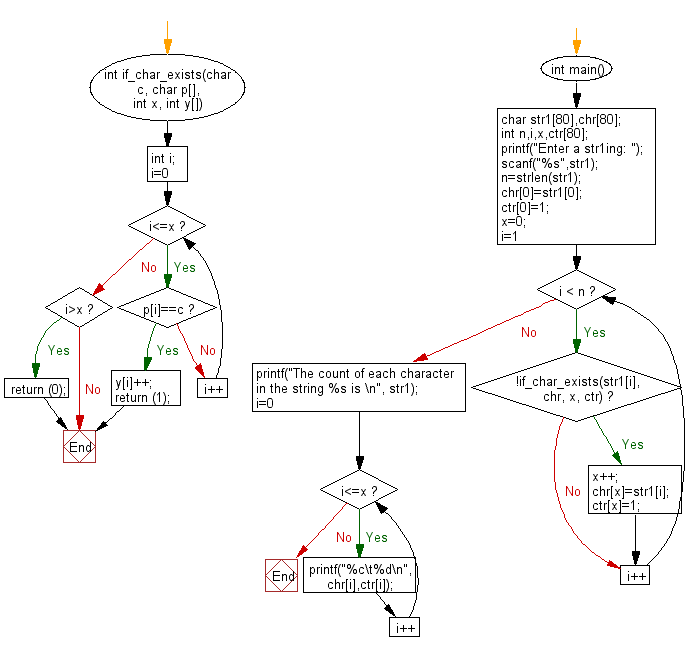﻿ C exercises: Count of each character in a given string - w3resource# C Programming: Count of each character in a given string

## C String: Exercise-33 with Solution

Write a C programming to count of each character in a given string.

Sample Solution:

C Code:

``````#include<stdio.h>
#include<string.h>
int if_char_exists(char c, char p[],  int x, int y[])
{
int i;
for (i=0; i<=x;i++)
{
if (p[i]==c)
{
y[i]++;
return (1);
}
}
if(i>x) return (0);
}
int main()
{
char str1,chr;
int n,i,x,ctr;
printf("Enter a str1ing: ");
scanf("%s",str1);
n=strlen(str1);
chr=str1;
ctr=1;
x=0;
for(i=1;i < n;  i++)
{
if(!if_char_exists(str1[i], chr, x, ctr))
{
x++;
chr[x]=str1[i];
ctr[x]=1;
}
}
printf("The count of each character in the string %s is \n", str1);
for (i=0;i<=x;i++)
printf("%c\t%d\n",chr[i],ctr[i]);
}
```
```

Sample Output:

``` Enter a str1ing: The count of each character in the string w3resource is
w	1
3	1
r	2
e	2
s	1
o	1
u	1
c	1
```

Flowchart :C Programming Code Editor:

Improve this sample solution and post your code through Disqus.

What is the difficulty level of this exercise?

﻿

## C Programming: Tips of the Day

How to measure time in milliseconds using ANSI C?

There is no ANSI C function that provides better than 1 second time resolution but the POSIX function gettimeofday provides microsecond resolution. The clock function only measures the amount of time that a process has spent executing and is not accurate on many systems.

You can use this function like this:

```structtimevaltval_before, tval_after, tval_result;

gettimeofday(&tval_before, NULL);

// Some code you want to time, for example:
sleep(1);

gettimeofday(&tval_after, NULL);

timersub(&tval_after, &tval_before, &tval_result);

printf("Time elapsed: %ld.%06ld\n", (long int)tval_result.tv_sec, (long int)tval_result.tv_usec);
```

This returns Time elapsed: 1.000870 on my machine.

Ref : https://bit.ly/3lpwoMn